Next: Other hidden Markov model Up: Previous hybrid models Previous: Previous hybrid models   Contents

## Switching state-space models

Figure 4.3: Bayesian network representations of some switching state-space model architectures. The round nodes represent Gaussian variables and the square nodes are discrete. The shaded nodes are observed while the white ones are hidden.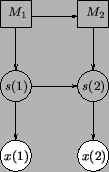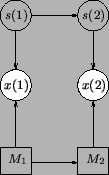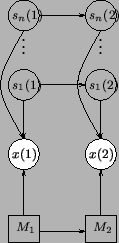Switching dynamics Switching observations Independent dynamical models 

There are several possible architectures for switching SSMs. Figure 4.3 shows some of the most basic ones . The first subfigure corresponds to the case where the function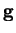and possibly the model for noise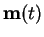in Equation (4.13) are different for different states. In the second subfigure, the function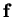and the noise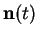depend on the switching variable. Some combination of these two approaches is of course also possible. The third subfigure shows an interesting architecture proposed by Ghahramani and Hinton  in which there are several completely separate SSMs and the switching variable chooses between them. Their model is especially interesting as it uses ensemble learning to infer the model parameters.

One of the problems with switching SSMs is that the exact E-step of the EM algorithm is intractable, even if the individual continuous hidden states are Gaussian. Assuming the HMM has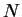states, the posterior of a single state variable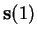will be a mixture ofGaussians, one for each HMM state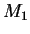. When this is propagated forward according to the dynamical model, the mixture grows exponentially as the number of possible HMM state sequences increases. Finally, when the full observation sequence of length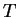is taken into account, the posterior of each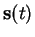will be a mixture of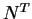Gaussians.

Ensemble learning is a very useful method in developing a tractable algorithm for the problem, although there are other heuristic methods for the same purpose. The other methods typically use some greedy procedure in collapsing the distribution and this may cause inaccuracies. This is not a problem with ensemble learning -- it considers the whole sequence and minimises the Kullback-Leibler divergence, which in this case has no local minima.Next: Other hidden Markov model Up: Previous hybrid models Previous: Previous hybrid models   Contents
Antti Honkela 2001-05-30